﻿ Variance Estimation Using Quartiles and their Functions of an Auxiliary Variable Scientific & Academic Publishing: The article detailed informationInternational Journal of Statistics and Applications

p-ISSN: 2168-5193    e-ISSN: 2168-5215

2012;  2(5): 67-72

doi: 10.5923/j.statistics.20120205.04

### Variance Estimation Using Quartiles and their Functions of an Auxiliary Variable

J. Subramani , G. Kumarapandiyan

Department of Statistics, Ramanujan School of Mathematical Sciences, Pondicherry University, R V Nagar, Kalapet, Puducherry, 605014, India

Correspondence to: J. Subramani , Department of Statistics, Ramanujan School of Mathematical Sciences, Pondicherry University, R V Nagar, Kalapet, Puducherry, 605014, India.
 Email: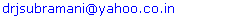Abstract

In this paper we have proposed a class of modified ratio type variance estimators for estimation of population variance of the study variable using Quartiles and their functions of the auxiliary variable are known. The biases and mean squared errors of the proposed estimators are obtained and also derived the conditions for which the proposed estimators perform better than the traditional ratio type variance estimator and existing modified ratio type variance estimators. Further we have compared the proposed estimators with that of traditional ratio type variance estimator and existing modified ratio type variance estimators for certain known populations. From the numerical study it is observed that the proposed estimators perform better than the traditional ratio type variance estimator and existing modified ratio type variance estimators.

Keywords: Inter-Quartile Range, Natural Populations, Simple Random Sampling, Semi-Quartile Average, Semi-Quartile Range

Cite this paper: J. Subramani , G. Kumarapandiyan , "Variance Estimation Using Quartiles and their Functions of an Auxiliary Variable", International Journal of Statistics and Applications, Vol. 2 No. 5, 2012, pp. 67-72. doi: 10.5923/j.statistics.20120205.04.

### 1. Introduction

Consider a finite population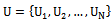ofdistinct and identifiable units. Let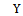be a real variable with value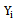measured on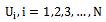giving a vector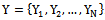. The problem is to estimate the populations mean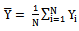or population variance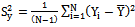on the basis of a random sample selected from the population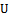. Estimating the finite population variance has great significance in various fields such as Industry, Agriculture, Medical and Biological Sciences. For example in matters of health, variations in body temperature, pulse beat and blood pressure are the basic guides to diagnosis where prescribed treatment is designed to control their variation. In this paper we intend to suggest some estimators for population variance. When there is no additional information on the auxiliary variable available, the simplest estimator of population variance is the simple random sample variance without replacement. It is common practice to use the auxiliary variable for improving the precision of the estimate of a parameter. In this paper, we consider the auxiliary information to improve the efficiency of the estimation of population variance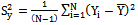in simple random sampling. When the information on an auxiliary variable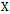is known, a number of estimators such as ratio, product and linear regression estimators are proposed in the literature.
When the correlation between the study variable and the auxiliary variable is positive, ratio method of estimation is quite effective. On the other hand, when the correlation is negative, Product method of estimation can be employed effectively. Among the estimators mentioned above, the ratio estimator and its modifications are widely used for the estimation of the variance of the study variable.
Estimation of population variance is considered by Isaki where ratio and regression estimators are proposed. Prasad and Singh have considered a ratio type estimator for estimation of population variance by improving Isaki estimator with respect to bias and precision. Arcos et al. have introduced another ratio type estimator, which has also improved the Isaki estimator, which is almost unbiased and more precise than the other estimators.
Before discussing further about the traditional ratio type variance estimator, existing modified ratio type variance estimators and the proposed modified ratio type variance estimators, the notations to be used in this paper are described below: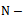Population size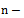Sample size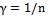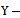Study variable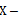Auxiliary variable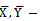Population means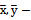Sample means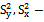Population variances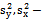Sample variances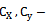Coefficient of variations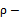Coefficient of correlation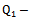First (lower) quartile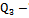Third (upper) quartile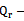Inter-quartile range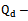Semi-quartile range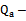Semi-quartile average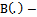Bias of the estimator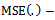Mean squared error of the estimator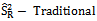ratio type variance estimator of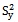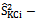Existing modified ratio type variance estimator of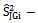Proposed modified ratio type variance estimator ofIsaki suggested a ratio type variance estimator for the population variancewhen the population variance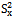of the auxiliary variableis known together with its bias and mean squared error and are as given below: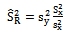(1)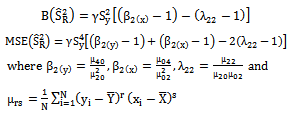The Ratio type variance estimator given in (1) is used to improve the precision of the estimate of the population variance compared to simple random sampling when there exists a positive correlation betweenand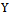. Further improvements are also achieved on the classical ratio estimator by introducing a number of modified ratio estimators with the use of known parameters like Co-efficient of Variation, Co-efficient of Kurtosis. The problem of constructing efficient estimators for the population variance has been widely discussed by various authors such as Agarwal and Sithapit, Ahmed et al., Al-Jararha and Al-Haj Ebrahem, Arcos et al., Bhushan, Cochran, Das and Tripathi, Garcia and Cebrain, Gupta and Shabbir, Isaki, Kadilar and Cingi[11,12], Murthy, Prasad and Singh, Reddy, Singh and Chaudhary, Singh et al.[17,19], Upadhyaya and Singh and Wolter.
Motivated by Singh et al., Sisodia and Dwivedi, and Upadhyaya and Singh, Kadilar and Cingi suggested four ratio type variance estimators using known values of Co-efficient of variation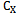and Co-efficient of Kurtosis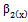of an auxiliary variabletogether with their biases and mean squared errors as given in the Table 1.
The existing modified ratio type variance estimators discussed above are biased but have minimum mean squared errors compared to the traditional ratio type variance estimator suggested by Isaki. The list of estimators given in Table 1 uses the known values of the parameters like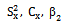and their linear combinations. Subramani and Kumarapandiyan used Quartiles and their functions of the auxiliary variable like Inter-quartile range, Semi-quartile range and Semi-quartile average to improve the ratio estimators in estimation of population mean. Further we know that the value of quartiles and their functions are unaffected by the extreme values or the presence of outliers in the population values unlike the other parameters like the variance, coefficient of variation and coefficient of kurtosis. The points discussed above have motivated us to introduce a modified ratio type variance estimators using the known values of the quartiles and their functions of the auxiliary variable.
Now briefly we will discuss about quartiles and its functions. The median divides the data into two equal sets. The first (lower) quartile is the middle value of the first set, where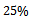of the values are smaller than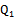andare larger. The third (upper) quartile is the middle value of the second set, where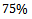of the values are smaller than the third quartile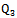andare larger. It should be noted that the median will be denoted by the notation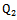, the second quartile. The inter-quartile range is another range used as a measure of the spread. The difference between upper and lower quartiles, which is called as the inter-quartile range, also indicates the dispersion of a data set. The formula for inter-quartile range is: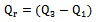(2)
The semi-quartile range is another measure of spread. It is calculated as one half of the differences between the quartilesand. The formula for semi-quartile range is: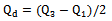(3)
Subramani and Kumarapandiyan suggested another new measure called as Semi-quartile average denoted by the notation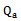and defined as: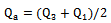(4)
 Table 1. Existing Modified ratio type variance estimators with their biases and mean squared errors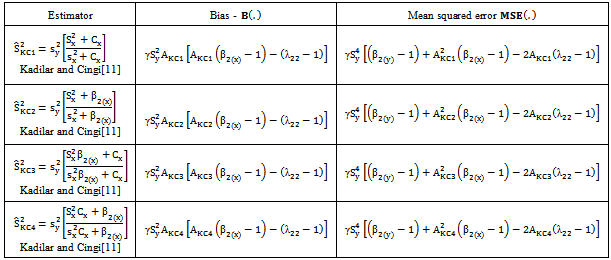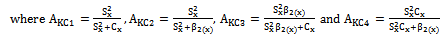### 2. Proposed Estimators Using Quartiles and Their Functions

In this section we have suggested a class of modified ratio type variance estimators using the quartiles and their functions of the auxiliary variable like Inter-quartile range, Semi-quartile range and Semi-quartile average
The proposed class of modified ratio type variance estimators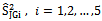for estimating the population variance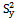together with the first degree of approximation, the biases and mean squared errors and the constants are given below:
 Table 2. Proposed modified ratio type variance estimators with their biases and mean squared errors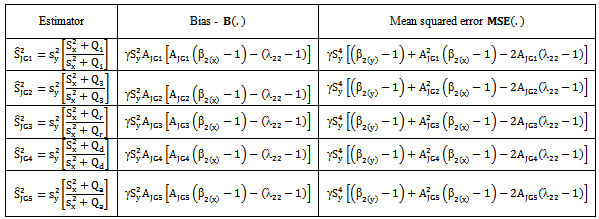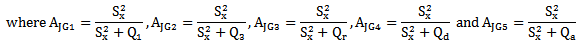### 3. Efficiency of the Proposed Estimators

As we mentioned earlier the bias and mean squared error of the traditional ratio type variance estimator are given below: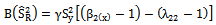(5)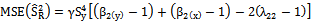(6)
For want of space; for the sake of convenience to the readers and for the ease of comparisons, the biases and mean squared errors of the existing modified ratio type variance estimators given in Table 1 are represented in single class as given below: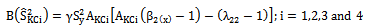(7)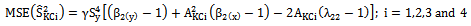(8)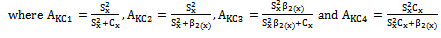In the same way, the biases and mean squared errors of the proposed modified ratio type variance estimators given in Table 2 are represented in a single class as given below: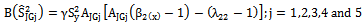(9)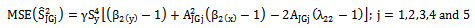(10)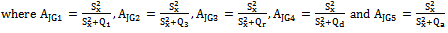From the expressions given in (6) and (10) we have derived the condition for which the proposed estimators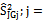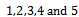are more efficient than the traditional ratio type variance estimator and it is given below: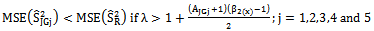(11)
From the expressions given in (8) and (10) we have derived the conditions for which the proposed estimators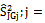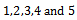are more efficient than the existing modified ratio type variance estimators given in Table 1,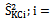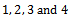and are given below: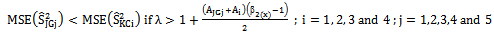(12)

### 4. Numerical Study

The performance of the proposed modified ratio type variance estimators are assessed with that of traditional ratio type estimator and existing modified ratio type variance estimators listed in Table 1 for certain natural populations. The populations 1 and 2 are the real data set taken from the Report on Waste 2004 drew up by the Italian bureau for the environment protection-APAT. Data and reports are available in the website http://www.osservatorionazionalerifiuti.it. In the data set, for each of the Italian provinces, three variables are considered: the total amount (tons) of recyclable-waste collection in Italy in 2003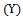, the total amount of recyclable-waste collection in Italy in 2002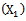and the number of inhabitants in 2003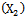. The population 3 is taken from Murthy given in page 228 and population 4 is taken from Singh and Chaudhary given in page 108. The population parameters and the constants computed from the above populations are given below:
 Table 3. Parameters and Constants of the Populations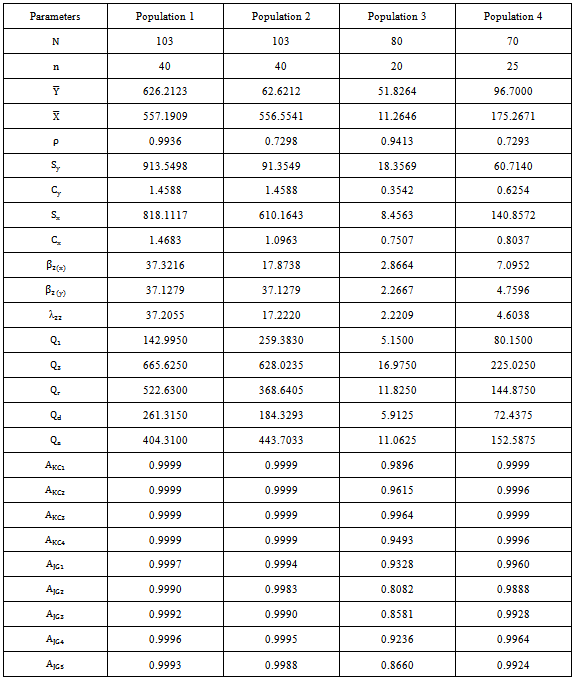The biases and mean squared errors of the existing and proposed modified ratio type variance estimators for the populations given above are given in the following Tables:
 Table 4. Biases of the existing and proposed modified ratio type variance estimators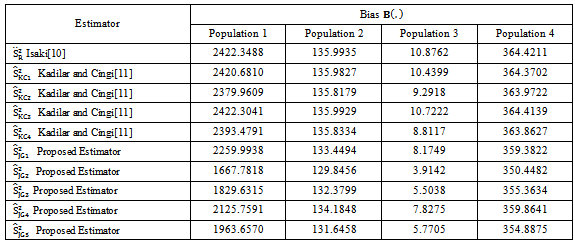Table 5. Mean squared errors of the existing and proposed modified ratio type variance estimators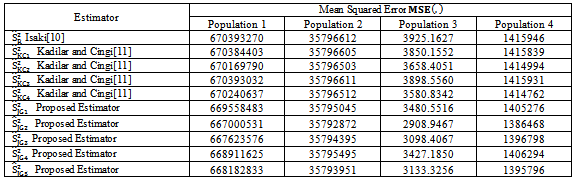From the values of Table 4, it is observed that the biases of the proposed modified ratio type variance estimators are less than the biases of the traditional and existing modified ratio type variance estimators. Similarly from the values of Table 5, it is observed that the mean squared errors of the proposed modified ratio type variance estimators are less than the mean squared errors of the traditional and existing modified ratio type variance estimators.

### 5. Conclusions

Estimating the finite population variance has great significance in various fields such as Industry, Agriculture, Medical and Biological sciences, etc. In this paper we have proposed a class of modified ratio type variance estimators using the quartiles and their functions of the auxiliary variable like Inter-quartile range, Semi-quartile range and Semi-quartile average. The biases and mean squared errors of the proposed modified ratio type variance estimators are obtained and compared with that of traditional ratio type variance estimator and existing modified ratio type variance estimators. Further we have derived the conditions for which the proposed estimators are more efficient than the traditional and existing estimators. We have also assessed the performance of the proposed estimators for some known natural populations. It is observed that the biases and mean squared errors of the proposed estimators are less than the biases and mean squared errors of the traditional and existing modified estimators for certain known populations. Hence we strongly recommend that the proposed modified ratio type variance estimator may be preferred over the traditional ratio type variance estimator and existing modified ratio type variance estimators for the use of practical applications.

### ACKNOWLEDGEMENTS

The second author wishes to acknowledge his sincere thanks to the Vice Chancellor, Pondicherry University for the financial support to carry out this research work through the University Fellowship.

### References

  Agarwal, M.C. and Sithapit, A.B. (1995). Unbiased ratio type estimation, Statistics and Probability Letters 25, 361-364  Ahmed, M.S., Raman, M.S. and Hossain, M.I. (2000). Some competitive estimators of finite population variance Multivariate Auxiliary Information, Information and Management Sciences, Volume11 (1), 49-54  Al-Jararha, J. and Al-Haj Ebrahem, M. (2012). A ratio estimator under general sampling design, Austrian Journal of Statistics, Volume 41(2), 105-115  Arcos, A., Rueda, M., Martinez, M.D., Gonzalez, S., Roman, Y. (2005). Incorporating the auxiliary information available in variance estimation, Applied Mathematics and Computation 160, 387-399  Bhushan, S. (2012). Some efficient sampling strategies based on ratio type, estimator, Electronic Journal of Applied Statistical Analysis, Volume 5(1), 74-88  Cochran, W. G. (1977). Sampling techniques, Third Edition, Wiley Eastern Limited  Das, A.K. and Tripathi, T.P. (1978). Use of auxiliary information in estimating the finite population variance, Sankhya 40, 139-148  Garcia, M.K. and Cebrain, A.A. (1997). Variance estimation using auxiliary information: An almost unbiased multivariate ratio estimator, Metrika 45, 171-178  Gupta, S. and Shabbir, J. (2008). Variance estimation in simple random sampling using auxiliary information, Hacettepe Journal of Mathematics and Statistics, Volume 37, 57-67  Isaki, C.T. (1983). Variance estimation using auxiliary information, Journal of the American Statistical Association 78, 117-123  Kadilar, C. and Cingi, H. (2006). Improvement in variance estimation using auxiliary information, Hacettepe Journal of Mathematics and Statistics Volume 35 (1), 111-115  Kadilar, C. and Cingi, H. (2006). Ratio estimators for population variance in simple and stratified sampling, Applied Mathematics and Computation 173, 1047-1058  Murthy, M.N. (1967). Sampling theory and methods, Statistical Publishing Society, Calcutta, India  Prasad, B. and Singh, H.P. (1990). Some improved ratio type estimators of finite population variance in sample surveys, Communication in Statistics: Theory and Methods 19, 1127-1139  Reddy, V.N. (1974). On a transformed ratio method of estimation, Sankhya, Volume C36, 59-70  Singh, D. and Chaudhary, F.S. (1986). Theory and analysis of sample survey designs, New Age International Publisher  Singh, H.P., Chandra, P. and Singh, S. (2003). Variance estimation using multi-auxiliary information for random non-response in survey sampling, STATISTICA, anno LXIII, n. 1, 23-40  Singh, H.P., Tailor, R., Tailor, R. and Kakran, M.S. (2004). An improved estimator of population mean using power transformation, Journal of the Indian Society of Agricultural Statistics 58(2), 223-230  Singh, H.P., Upadhyaya, U.D. and Namjoshi, U.D. (1988). Estimation of finite population variance, Current Science 57, 1331-1334  Sisodia, B.V.S. and Dwivedi, V.K. (1981). A modified ratio estimator using coefficient of variation of auxiliary variable, Journal of the Indian Society of Agricultural Statistics 33(1), 13-18  Subramani, J. and Kumarapandiyan, G. (2012). Modified ratio estimators for population mean using function of quartiles of auxiliary variable, Bonfring International Journal of Industrial Engineering and Management Science, Vol. 2(2), 19-23  Upadhyaya, L.N. and Singh, H.P. (1999). Use of transformed auxiliary variable in estimating the finite population mean, Biometrical Journal 41 (5), 627-636  Upadhyaya, L. N. and Singh, H. P. (2006). Almost unbiased ratio and product-type estimators of finite population variance in sample surveys, Statistics in Transition 7 (5), 1087–1096  Wolter, K.M. (1985). Introduction to Variance Estimation, Springer-Verlag  http://www.osservatorionazionalerifiuti.it/ElencoDocPub.asp?A_TipoDoc=6